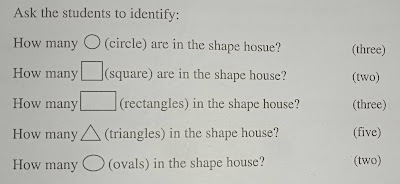# Geometry (Basic Shapes from real life objects)

Lesson Plan of Geometry (Basic Shapes from real life objects)

Subject Mathematics

Students` Learning Outcomes

• Recognize and match objects, from daily life of similar shape.
• Identify the following basic shapes: as;
• Rectangle, square, circle, oval, and triangle
• Identify the basic shapes from real life objects
• Match similar basic shapes

Information for Teachers

• Geometry is a kind of mathematics that studies the size, shapes, and positions of things. There are flat shapes and solid shapes in geometry, squares, circles and triangles are some of the simplest shapes.
• Geometry can help people find: as;
• The surface area of a house, so they can buy the right amount of paint
• The volume of box, to see if it is big enough to hold a liter of food
• The area of the form, so it can be divided into equal parts
•

• While teaching the lesson, the teacher should also consult textbook at all steps wherein and whenever it is applicable.

Material / Resources

Writing board, chalk/marker, cut-outs of shapes (square, triangle, and circle) in different colors and size, poster of shapes house

Introduction

• Tell the students that shapes are the outer form or appearance of something. Ask them find some similar shapes from their classroom.
• Make a circle with their hand and ask /tell them that this is a circle.
• Ask students to make the shape using their hands too
• Ask them to find other objects in the class that ar circles. Such as sharpeners, watch dial, hair clips
• Brainstorm on other things that are shaped like a circle
• Then make a square with your hand and ask students to do the same
• Again ask them to look for more objects of that shape in the classroom
 Activity 1 Demonstrate how we find the similar shapes of different objects by showing te cut outs of shapes (square, rectangle, and circle) having different size and colors. Ask the students to make the pairs of different objects that have shapes. (Coins and clock, window and board, ball and football, ball and orange, etc. Collect students` responses and make pairs of different objects for same shapes on the board.

 Activity 2 Demonstrate that shapes have their names by showing cut-outs of square, circle, rectangle, triangle, and oval to the students. Paste /draw these shapes on the board and label them. Ask the students to find out shapes of different objects in the classroom as “square, circle, rectangle, triangle, and oval shapes”

 Activity 3 Hang the shape house poster in front of class or draw the following sketch on the board.Activity 4 Ask the students` to match shapes of different objects using worksheets given on textbook. Collect their responses  and guide them

Sum up / Conclusion
• Square, rectangle, triangle, circle and oval are basic shapes.

Assessment

• Draw square, circle, rectangle, on the board and ask different students to identify
• Make the shapes using your hands and ask students to guess.
• Teacher is also required to involve the students in solving the problems given in the exercise at the end of unit/chapter.